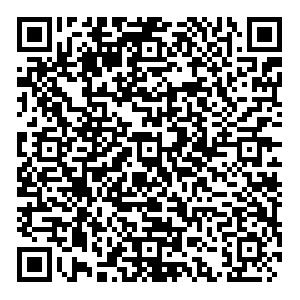# Relative probabilities of breakup channels in reactions of 6,7Li with 209Bi at energies around and above the Coulomb barrier

• Coincidence measurements of breakup fragments in reactions of ${^{6, 7}{\rm{Li}}}$with ${^{209}{\rm{Bi}}}$at energies around and above the Coulomb barrier were carried out using a large solid-angle covered detector array. Through the Q values along with the relative energies of the breakup fragments, different breakup components (prompt breakups and delayed breakups) and different breakup modes ($\alpha + t$, $\alpha + d$, $\alpha + p$, and $\alpha + \alpha$) are distinguished. A new breakup mode, $\alpha + t$, is observed in ${^{6}{\rm{Li}}}$-induced reactions at energies above the Coulomb barrier. Correlations between breakup modes and breakup components as well as their variations with the incident energy are investigated. The results will help us better understand the breakup effects of weakly bound nuclei on the suppression of a complete fusion, particularly for the above-barrier energies.
••Get Citation
Yong-Jin Yao, Cheng-Jian Lin, Lei Yang, Nan-Ru Ma, Dong-Xi Wang, Gao-long Zhang, Guang-Xin Zhang, Hui-Ming Jia, Feng Yang, Fu-Peng Zhong, Pei-Wei Wen, Xiu-Bo Qin and Hong-Ming Zhao. Relative probabilities of breakup channels in reactions of 6,7Li with 209Bi at energies around and above the Coulomb barrier[J]. Chinese Physics C.
Yong-Jin Yao, Cheng-Jian Lin, Lei Yang, Nan-Ru Ma, Dong-Xi Wang, Gao-long Zhang, Guang-Xin Zhang, Hui-Ming Jia, Feng Yang, Fu-Peng Zhong, Pei-Wei Wen, Xiu-Bo Qin and Hong-Ming Zhao. Relative probabilities of breakup channels in reactions of 6,7Li with 209Bi at energies around and above the Coulomb barrier[J]. Chinese Physics C.Milestone
Received: 2020-12-29
Article Metric

Article Views(101)
PDF Downloads(14)
Cited by(0)
Policy on re-use
To reuse of subscription content published by CPC, the users need to request permission from CPC, unless the content was published under an Open Access license which automatically permits that type of reuse.
###### 通讯作者: 陈斌, bchen63@163.com
• 1.

沈阳化工大学材料科学与工程学院 沈阳 110142

Title:
Email:

## Relative probabilities of breakup channels in reactions of 6,7Li with 209Bi at energies around and above the Coulomb barrier

###### Corresponding author: Gao-long Zhang, zgl@buaa.edu.cn
• 1. State Key Laboratory of Software Development Environment, Beihang University, Beijing 100191, China
• 2. School of Physics, Beihang University, Beijing 100191, China
• 3. China Institute of Atomic Energy, Beijing 102413, China
• 4. Guangxi Normal University, Guangxi 541004, China
• 5. X Lab, the Second Academy of CASIC, Beijing 100854, China

Abstract: Coincidence measurements of breakup fragments in reactions of ${^{6, 7}{\rm{Li}}}$with ${^{209}{\rm{Bi}}}$at energies around and above the Coulomb barrier were carried out using a large solid-angle covered detector array. Through the Q values along with the relative energies of the breakup fragments, different breakup components (prompt breakups and delayed breakups) and different breakup modes ($\alpha + t$, $\alpha + d$, $\alpha + p$, and $\alpha + \alpha$) are distinguished. A new breakup mode, $\alpha + t$, is observed in ${^{6}{\rm{Li}}}$-induced reactions at energies above the Coulomb barrier. Correlations between breakup modes and breakup components as well as their variations with the incident energy are investigated. The results will help us better understand the breakup effects of weakly bound nuclei on the suppression of a complete fusion, particularly for the above-barrier energies.

### HTMLII.   EXPERIMENTAL SETUPIII.   DATA ANALYSISIV.   RELATIVE PROBABILITIES OF BREAKUP CHANNELS
Reference (24)
PDF查看关注分享

Top

### 目录/DownLoad:  Full-Size Img  PowerPoint